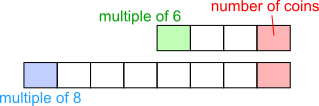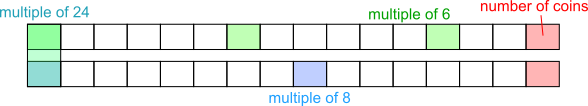#### You may also like### N000ughty Thoughts

How many noughts are at the end of these giant numbers?### Mod 3

Prove that if a^2+b^2 is a multiple of 3 then both a and b are multiples of 3.### Novemberish

a) A four digit number (in base 10) aabb is a perfect square. Discuss ways of systematically finding this number. (b) Prove that 11^{10}-1 is divisible by 100.

# Coin Collection

##### Age 14 to 16 ShortChallenge Level

Counting backwardsMultiples of both 6 and 8 are multiples of 24

Using number facts
The number of coins in Tom's collection is 3 more than a multiple of 6 and also 7 more than a multiple of 8. The smallest number that satisfies both conditions is 15. The lowest common multiple of 6 and 8 is 24, so the conditions will also be met by numbers that exceed 15 by a multiple of 24, that is 39, 63, 87, etc. So when Tom puts his coins in piles of 24, 15 remain.

This problem is taken from the UKMT Mathematical Challenges.
You can find more short problems, arranged by curriculum topic, in our short problems collection.